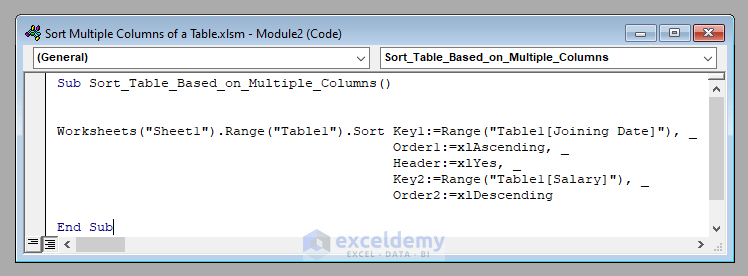# How to Sort Multiple Columns of a Table with Excel VBA (2 Methods)

Get FREE Advanced Excel Exercises with Solutions!

In this article, I’ll show you how you can sort multiple columns of a table using VBA in Excel. You’ll learn to sort all the columns of a table based on both a single column and multiple columns.

Sort Multiple Columns of a Table with Excel VBA (Quick View)

``````Sub Sort_Table_Based_on_Multiple_Columns()

Worksheets("Sheet1").Range("Table1").Sort Key1:=Range("Table1[Joining Date]"), _
Order1:=xlAscending, _
Key2:=Range("Table1[Salary]"), _
Order2:=xlDescending

End Sub``````

## 2 Suitable Ways to Sort Multiple Columns of a Table with Excel VBA

Here we’ve got a table called Table1 in a worksheet called Sheet1 of an Excel workbook that contains three columns namely Employee Name, Joining Date, and Salary.Our objective today is to sort multiple columns of this table with Excel VBA.

### 1. Sort Multiple Columns of a Table based on a Single Column with Excel VBA

First of all, we’ll sort all the columns of this table based on a single column.

Let’s try to sort the whole table (Table1) of Sheet1 according to the ascending order of the joining dates of the employees (Seniority-wise).

⧭ Overview of the Code:

Before going to the main code, let’s have an overview of the code first. We’ll use the Sort method of VBA for this purpose.

The Sort method of VBA takes 3 parameters.

⧪ Key: The column based on which sorting will be made. Here it’s Table1[Joining Date].

⧪ Order: The order in which the Key column will be sorted. Here it’s xlAscending.

⧪ Header: Represents whether the table has headers or not. Here it’s xlYes (As the table has headers).

⧭ Special Note:

The Key parameter and the Order parameter can be more than one in number (in case the table is sorted based on multiple columns). So we have to number them in the order, like Key1, Order1, Key2, Order2, etc.

Here we have only one Key and one Order. So we’ll use only Key1 and Order1.

⧭ VBA Code:

Now it’s the time for the code. The complete VBA code will be:

``````Sub Sort_Table_Based_on_Single_Column()

Worksheets("Sheet1").Range("Table1").Sort Key1:=Range("Table1[Joining Date]"), _
Order1:=xlAscending, _

End Sub``````⧭ Output:

Run the code (Obviously after changing the inputs according to your need). You’ll find the whole table sorted according to the ascending order of the joining dates of the employees.### 2. Sort Multiple Columns of a Table based on Multiple Columns with Excel VBA

In the previous method, we sorted the table based on the joining dates only.

But on the joining dates, there are some repetitions. That is, the joining dates of a few employees are the same. This time when such a case occurs, we’ll sort them in the descending order of their salaries.

Therefore, the summary is, first, we’ll sort based on the joining dates (ascending order), then based on salaries (descending order).

⧭ Overview of the Code:

We’ll again use the Sort method of VBA. But this time, there will be 2 Key parameters (Key1:= Table1[Joining Date] and Key2:= Table1[Salary]) and 2 Order parameters (Order1:= xlAscending and Order2:= xlDescending)

⧭ VBA Code:

Therefore, the VBA code will be:

``````Sub Sort_Table_Based_on_Multiple_Columns()

Worksheets("Sheet1").Range("Table1").Sort Key1:=Range("Table1[Joining Date]"), _
Order1:=xlAscending, _
Key2:=Range("Table1[Salary]"), _
Order2:=xlDescending

End Sub``````⧭ Output:

Run the code (After changing the inputs). This time you’ll find the whole table sorted according to the ascending order of the joining dates of the employees first, then according to the descending order of the salaries.## Things to Remember

Here I’ve used the Sort method of VBA to sort the table. But there is a built-in function in Excel called the Sort function that we can use directly to sort any range (Available in Office 365 only).

## Conclusion

So these are the methods to sort multiple columns of a table using VBA in Excel. Do you have any questions? Feel free to ask us. And don’t forget to visit our site ExcelDemy for more posts and updates.

## What is ExcelDemy?

ExcelDemy Learn Excel & Excel Solutions Center provides free Excel tutorials, free support , online Excel training and Excel consultancy services for Excel professionals and businesses. Feel free to contact us with your Excel problems.

Tags:Rifat Hassan

Hello! Welcome to my profile. Here I will be posting articles related to Microsoft Excel. I am a passionate Electrical Engineer holding a Bachelor’s degree in Electrical and Electronic Engineering from the Bangladesh University of Engineering and Technology. Besides academic studies, I always love to keep pace with the revolution in technology that the world is rushing towards day by day. I am diligent, career-oriented, and ready to cherish knowledge throughout my life.

1 Comment
1. ReplyThank you for such brilliant code.Advanced Excel Exercises with Solutions PDF